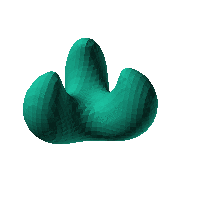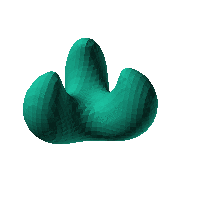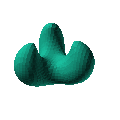Blob Farm# blob08The blob in all its glory:This is based on the monkey saddle formula.

$\frac{x\left({x}^{2}-3{y}^{2}\right)+0.6\text{}{z}^{3}+{z}^{2}-12z}{{\left({\left({x}^{2}+{y}^{2}+{z}^{2}\right)}^{3/2}-20\sqrt{{x}^{2}+{y}^{2}+1.\text{}{z}^{2}}+50\right)}^{2}}>0.01$Click on the snapshot to download the blob's stl file.Octave Code:
```1; # Prevent Octave from thinking that this is a function
# though one is defined here

function w = f(x2,y2,z2,c,r,e)
x  = (x2-c(1))/r(1);
y  = (y2-c(2))/r(2);
z  = (z2-c(3))/r(3);
# function at origin must be <0, and >0 far enough away.  w=0 defines the surface
w = (25+4*x.^2+-1*x.^3+4*y.^2+3* ...
x.*y.^2+12*z+3*z.^2+-0.6*z.^3+1*(x.^2+y.^2+ ...
z.^2).^(3/2)+0.01*(x.^2+y.^2+z.^2).^3+-20*(x.^2+y.^2+ ...
z.^2).^(1/2)+-0.4*(x.^2+y.^2+z.^2).^(3/2).*(x.^2+y.^2+ ...
z.^2).^(1/2));

endfunction;

```
GNU Octave